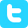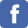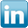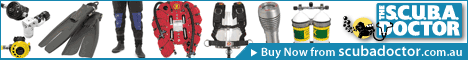search
> contact Lloyd
> Lloyd's blog
> forums# Equivalent Narcotic Depth (END) Calculator for Trimix and Heliox

Warning: This END calculator is for aiding in the calculation of decompression obligations when diving using Trimix or Heliox. It is not suitable for calculating narcotic effects and decompression requirements when using Nitrox.

Disclaimer: I take no responsibility for the accuracy of the calculations and data below. Always verify against other sources.

This calculator can be used to find the equivalent narcotic depth (END) in metres and feet of the provided fraction of helium (FHe) at various planned diving depths in metres when using Trimix or Heliox. Just enter values for "Fraction of Helium (FHe)" and "Planned Depth" and click on the "Submit" button.

END Calculator
* Indicates required field
 *Fraction of Helium (FHe) e.g. 1=100%, 0.4=40% etc. *Planned Depth e.g. 60 metres

Diving a Fraction of Helium (FHe) of .4 or 40%
at a Planned Depth of 60 metres (196.9 feet)
your Equivalent Narcotic Depth (END) rounding deeper is:

## 32.0 metres

in imperial units:

## 105 feet

Disclaimer:
This calculator provides information about gas use in scuba diving. UNDER NO CIRCUMSTANCES is there any implication that any gas mixture is safe or even life supporting at any depth. If you have not recived training in technical scuba diving, Nitrox, Trimix or Heliox you should not attempt to use any of these results. Improper use of gasses in diving can result in injury or DEATH.

## Explanation

We have to know our maximum operating depth (MOD) when we dive using Trimix or Heliox breathing gas mixtures that contain Helium (He), Nitrogen (N2) and Oxygen (O2) in different proportions to those in air.

If we have access to Trimix or Heliox tables there is not that much more to think about. However, if we only have air tables we have to calculate the equivalent narcotic depth (END) in order to be able to determine the approximate decompression requirements for the dive.

The equivalent narcotic depth (END) for a given Trimix or Heliox mix and planned diving depth, is the depth of a dive when breathing air (i.e. FO2 = .21, O2% = 21%, EAN21) that would have the same partial pressure of nitrogen (ppN2) and Oxygen (ppO2) as the breathing gas in question. For example, a trimix containing 20% oxygen, 40% helium, 40% nitrogen (trimix 20/40) being used at 60 metres (197 ft) has an END of 32 metres (105 ft).

## Formulas

To calculate the equivalent marcotic depth (END) using Trimix or Heliox for a specific FHe and planned diving depth, the following formulas are used:

#### Metric - metres

END (m) = ((Depth + 10) x (1 - FHe)) - 10

In which Depth is the planned diving depth in metres, FHe is decimal value of the fraction of helium in the gas mixture. For example, if a gas contains 40% helium and the planned depth is 60 metres,
the END (m) is ((60 + 10) x (1 - .4)) - 10 = 32 metres.

#### Imperial - feet

END (ft) = ((Depth + 33) x (1 - FHe)) - 33

In which Depth is the planned diving depth in feet, FHe is decimal value of the fraction of helium in the gas mixture. For example, if a gas contains 40% helium and the planned depth is 197 feet,
the END (ft) is ((197 + 33) x (1 - .4)) - 33 = 105 feet.

[ previous | up | next ]